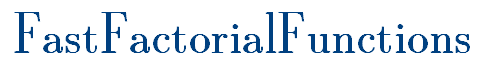N !There are five algorithms which everyone who wants to compute the factorial n! = 1.2.3...n should know. The algorithm , because it is simple and the fastest algorithm which does not use prime factorization. The algorithm , because it is the (asymptotical) fastest algorithm known to compute n!. The algorithm is based on the notion of the 'Swing Numbers' and computes n! via the prime factorization of these numbers. The ingenious algorithm of which uses only additions! Though of no practical importance (because it is slow), it has the fascination of an unexpected solution. The algorithm which uses no Big-Integer library and can be easily implemented in any computer language and is even fast up to 10000!. The algorithm, which is the PrimeSwing algorithm with improved performance using methods of concurrent programming and thus taking advantage of multiple core processors. If you do not attach great importance to high performance then get a BigInteger library and use: BigInt recfact(long start, long n) { long i; if (n <= 16) { BigInt r = new BigInt(start); for (i = start + 1; i < start + n; i++) r *= i; return r; } i = n / 2; return recfact(start, i) * recfact(start + i, n - i); } BigInt factorial(long n) { return recfact(1, n); }  And here is an algorithm which nobody needs, for the Simple-Minded only: long factorial(long n) {return n <= 1 ? 1 : n*factorial(n-1);} Just don't use it!

An example of a PrimeSwing computation:As this example shows an efficient computation of the factorial function reduces to an efficient computation of the swinging factorial n≀. Some information about these numbers can be found here and here. The prime factorization of the swing numbers is crucial for the implementation of the PrimeSwing algorithm.

A concise description of this algorithm is given in this write-up (pdf) and in the SageMath link below (Algo 5).

 Link Content Algorithms A very short description of 21 algorithms for computing the factorial function n!. X Julia factorial *NEW* The factorial function based on the swinging factorial which in turn is computed via prime factorization implemented in Julia. Mini Library The factorial function, the binomial function, the double factorial, the swing numbers and an efficient prime number sieve implemented in Scala and GO. Browse Code Various algorithms implemented inJava, C# and C++. SageMath Implementations in SageMath. LISP Implementations in Lisp. Benchmarks Benchmark 2013: With MPIR 2.6 you can calculate 100.000.000! in less than a minute provided you use one of the fast algorithms described here. Conclusions Which algorithm should we choose? Download Download a test application and benchmark yourself. X Approximations A unique collection! Approximation formulas. Gamma quot Bounds for Gamma(x+1)/Gamma(x+1/2) Gamma shift Why is Gamma(n)=(n-1)! and not Gamma(n)=n! ? X Hadamard Hadamard's Gamma function and a new factorial function [MathJax version] History Not even Wikipedia knows this! The early history of the factorial function. Notation On the notation n! Binary Split For coders only. Go to the page of the day. Sage / Python Implementation of the swing algorithm. ‼ Double Factorial The fast double factorial function. Prime Factorial Primfakultaet ('The Primorial', in German.) Bibliography Bibliography on Inequalities for the Gamma function. X Bernoulli &Euler Exotic Applications: Inclusions for the Bernoulli and Euler numbers. Binomial Fast Binomial Function (Binomial Coefficients). Variations A combinatorial generalization of the factorial. X Stieltjes' CF On Stieltjes' Continued Fraction for theGamma Function. al-Haytham /Lagrange The ignorance of some western mathematicians.A deterministic factorial primality test. Factorial Digits Number of decimal digits of 10n! Calculator Calculate n! for n up to 9.999.999.999 . RPN-Factorial The retro-factorial page! Permutations Awesome! Permutation trees, the combinatorics of n!. Perm. trees Download a pdf-poster with 120 permutation trees! Gamma LogGamma Plots of the factorial (gamma) function. External links Some bookmarks.

Fast-Factorial-Functions: The Homepage of Factorial Algorithms. (C) Peter Luschny, 2000-2017. All information and all source code in this directory is free under the Creative Commons Attribution-ShareAlike 3.0 Unported License. This page is listed on the famous "Dictionary of Algorithms and Data Structures" at the National Institute of Standards and Technology's web site (NIST). Apr. 2003 / Apr. 2017 : 800,000 visitors! Thank you!